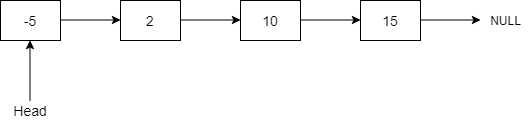# Remove All Nodes with Value K

Posted: 15 Mar, 2021
Difficulty: Easy

## PROBLEM STATEMENT

#### A singly linked list is a linear data structure in which we can traverse only in one direction i.e., from Head to Tail. It consists of several nodes where each node contains some data and a reference to the next node.##### Input format:
``````The first line contains an integer 'T' which denotes the number of test cases or queries to be run. Then, the T test cases follow.

The first line of each test case contains space-separated integers denoting the values of nodes of the Linked List. The Linked List is terminated with -1. Hence, -1 is never a node value of the Linked List.

The second line of each test case contains a single integer 'K' which denotes the value of the nodes to be removed.
``````
##### Output format:
``````For each test case, print the elements of the resultant Linked List after removing all nodes having the value 'K'.
``````
##### Note:
``````You do not need to print anything. It has already been taken care of. Just implement the given function.
``````
##### Constraints:
``````1 <= T <= 10
1 <= N <= 10^5
0 <= node.data <= 10^9 and node.data != -1
0 <= K <= 10^9

Where 'node.data' denotes the value of a Linked list node, and 'K' denotes the value of the nodes to be removed.

Time limit: 1 sec
``````Approach 1

Approach: The idea is to use recursion to solve the problem. The working of the recursive function is explained below. The basic idea is pretty simple that if a node has value K, then we will remove this node from the Linked List, and our output will be the node returned by the recursive call for its next node. Otherwise, our output will be the current node itself.

Working of the recursive function:

1. If the node ‘HEAD’ is a NULL node, then we will return the node ‘HEAD’.  This serves as the base case.
2. Let ‘TEMP’ be a node that stores the value returned by recursive call for the node ‘HEAD → NEXT'.
3. Update ‘HEAD -> NEXT' as ‘TEMP’, as ‘TEMP’ is the head of the Linked List after removing all occurrences of the value ‘K’.
4. If ‘HEAD → DATA' is equal to K, then we will return the node ‘HEAD → NEXT'. As we want the current node to be removed from the Linked List, so we are returning its next node as the head of the Linked List.
5. Otherwise, we will return the node ‘HEAD’.